# Polynomial Construction from Sequences V

Where we left off, we found a generalized formula for calculating any Nth order polynomial for any sequence of numbers. However, the method in which we organized the terms was grouping it by a common power of x. What if instead, we grouped them by a common CN?

Let's revisit the results of some of the generalized forms and do some regrouping. For making things slightly less verbose I'm going back to the letter variable notation for a moment.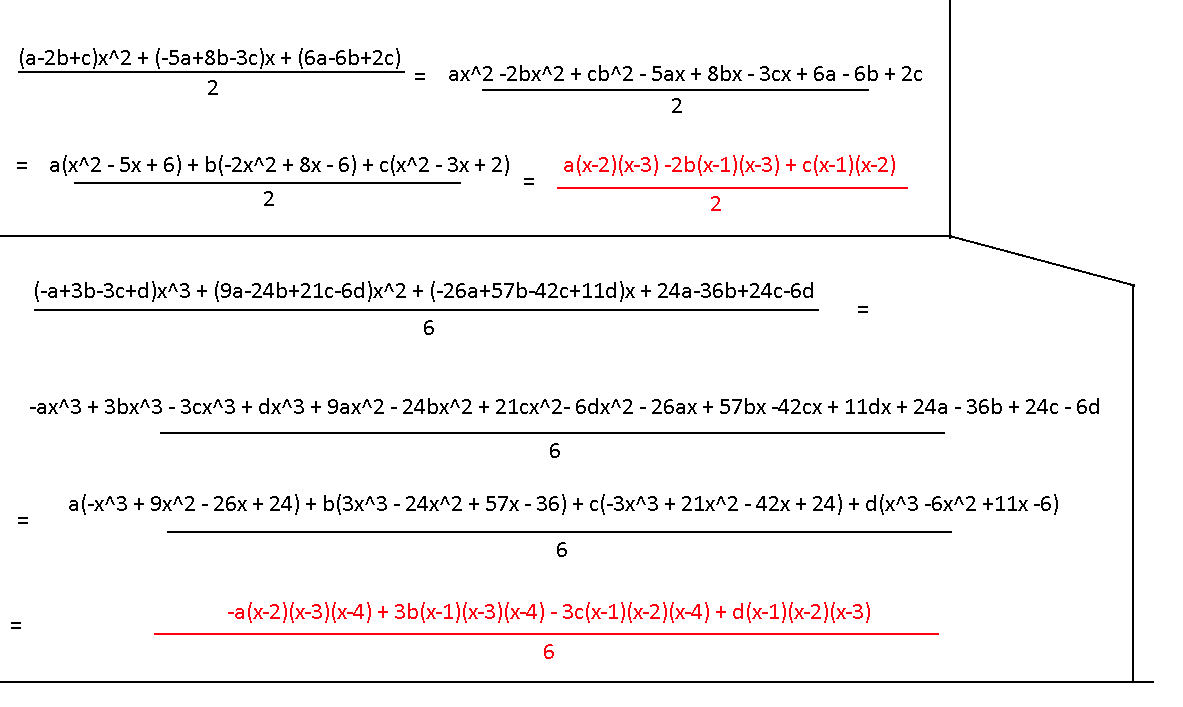At first it doesn't look any better than before, but if you try to factor as much of what's in the parentheses as possible, you end up with a shockingly nice form (the part in the red). That's not just a single term; those are the full expressions of the two-term and three-term sequence. It's time to get back to generalizing!

The nature of the letter terms are very familiar: alternating signs with the coefficients representing elements of Pascal’s triangle. We already know how to represent that. The slightly tricky part is going to be generalizing the x factors after every term, which is what the (???) part is in the image below.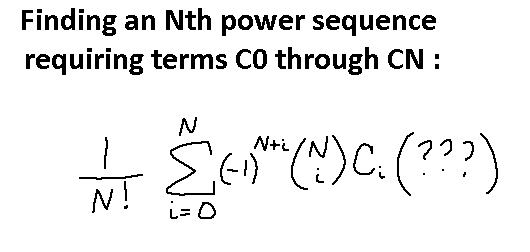If you take a look at just the x terms in the 3rd order expression, notice how there are exactly three of them for each term, and that each term is only missing one element out of a 4-element set of (x-1)(x-2)(x-3)(x-4). Specifically, the first term is missing the first element (x-1), the second term is missing the second element (x-2), and so on. Firstly, how do we even say we want (x-1)(x-2)(x-3)(x-4)? It's almost like (x-1)!, only all the terms cut off after (x-4). If there's one thing we learned from messing with factorials and the combination function, it's that if we put an (x-5)! in the denominator, it will cause all terms in (x-1)! to cancel out after (x-4). Then the only issue left is remembering to leave out the nth element on the nth term. Something like this should work.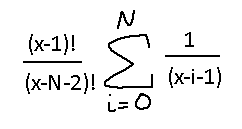We have these two separate, functional pieces. One of them calculates the CN term, and one calculates the factorial-like nature of the x factors. Time to combine them!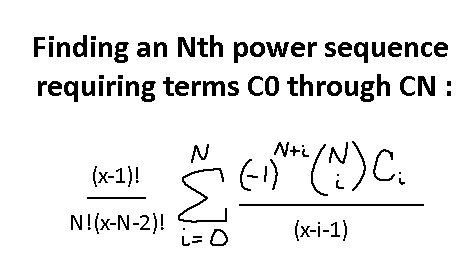That will give us a full Nth power formula for any N? Obviously we're going to test this. Unlike the last time, we're going big.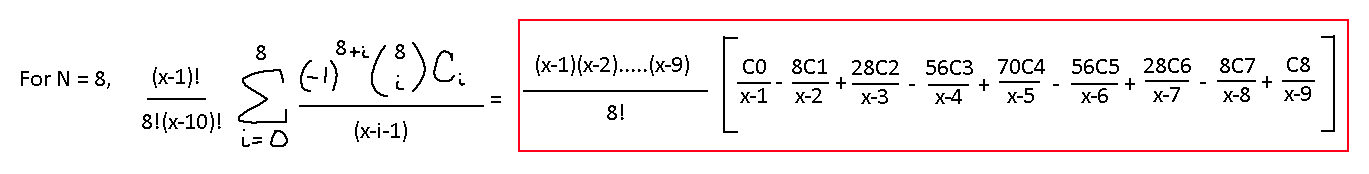If this is supposed to be the 8th power formula, then surely it can give me a polynomial whose first 8 terms in the sequence are 0 and the last term is a 1, right?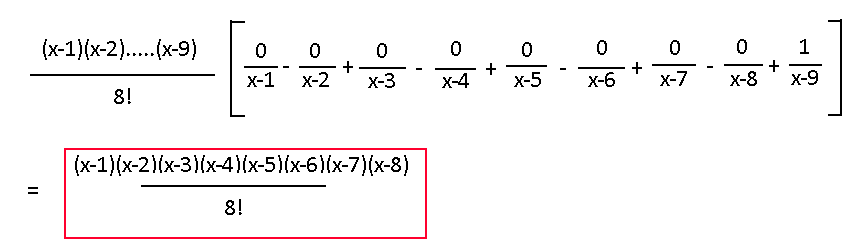…okay, I should've see that one coming. If you attempt to graph this function then it does indeed hit the x-axis for x = 1, 2, etc…, and then for less than x = 0 or greater than x = 10 the line just skyrockets upwards, as 8th order functions tend to do. All it took was a different method of organization to save orders of magnitudes of work.

Okay, NOW are we done? Well… we could stop here. See, this isn't a perfectly generalized solution. Why not? Well, we always assume for every sequence of numbers that we want those numbers mapped to x = 1, x = 2, x = 3, etc. What if I want to start at x = 0? What if I have just two values but I want them to exist at x = -1 and x = 4? Basically, what if I want to input a series of points (x0, y0), (x1, y1), etc. and have a formula that outputs a polynomial which passes through those points? If you think about it this way, we only solved a very specific set of cases for that, where we assumed (1, y0), (2, y1), (3, y2), etc. Oh no.

On the next post, we will rewrite our process from scratch to try and discover this truly generalized formula. We should have the knowledge and capability now to figure out what new processes will be necessary for this.